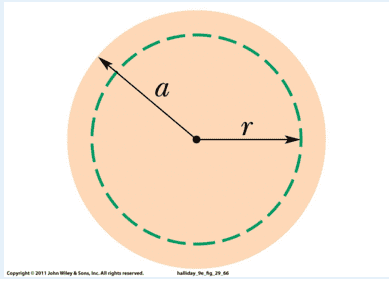# Biot-Savart's Law for cylindrical conductor

## Homework Statement

The figure shows a cross section across a diameter of a long cylindrical conductor of radius a = 2.92 cm carrying uniform current 151 A. What is the magnitude of the current's magnetic field at the center of the conductor?## Homework Equations

Biot-Savart's Law

## The Attempt at a Solution

B for circular loop = ui/2r
B = ui/2a
B = 0.0032 T
Which is the wrong answer, what happened?

TSny
Homework Helper
Gold Member
B for circular loop = ui/2r
This formula is for the magnetic field at the center of a circular loop of current. But in your problem the current is not flowing in a circular loop. It is flowing in a long, straight, cylindrical conductor.

This formula is for the magnetic field at the center of a circular loop of current. But in your problem the current is not flowing in a circular loop. It is flowing in a long, straight, cylindrical conductor.
Is this still do-able with the Biot Savart law?

TSny
Homework Helper
Gold Member
Yes, you can easily find B at the central axis by using the Biot-Savart law and symmetry.

However, if you want to find B for an arbitrary value of r in the picture, then it would be easier to use another law.

Yes, you can easily find B at the central axis by using the Biot-Savart law and symmetry.

However, if you want to find B for an arbitrary value of r in the picture, then it would be easier to use another law.
In this case, I think the current is flowing through the center. Then that means there is no field at the center right?

TSny
Homework Helper
Gold Member
In this case, I think the current is flowing through the center. Then that means there is no field at the center right?
I'm not understanding this argument. Can you elaborate? The current is flowing at all points of the cylinder, not just along the central axis.

I'm not understanding this argument. Can you elaborate? The current is flowing at all points of the cylinder, not just along the central axis.
My bad, then can you show me how to do this problem? We have only learned so far the law for wires.

TSny
Homework Helper
Gold Member
The problem only asks for the B field at the center axis of the cylinder. For this, you can just use symmetry arguments. Think of the total current distribution as made up of a lot of long, parallel, straight filaments of current. Each filament is like a thin, straight wire carrying current. Use what you know about the direction of the B field due to a long, straight wire.

The problem only asks for the B field at the center axis of the cylinder. For this, you can just use symmetry arguments. Think of the total current distribution as made up of a lot of long, parallel, straight filaments of current. Each filament is like a thin, straight wire carrying current. Use what you know about the direction of the B field due to a long, straight wire.
For parallel wires carrying the same current, there will be no net magnetic field at a point between them. So can I generalize this to the situation here?

TSny
Homework Helper
Gold Member
Yes. Good.

Yes. Good.
Now the problem asks for the field at radial distance 1 cm. How can I use symmetry for this?

TSny
Homework Helper
Gold Member
Now you need to do some math! The law of choice would be Ampere's law, not the Biot-Savart law. Symmetry will still be important.

Now you need to do some math! The law of choice would be Ampere's law, not the Biot-Savart law. Symmetry will still be important.
Edit: I will come back to this

Last edited:
Now you need to do some math! The law of choice would be Ampere's law, not the Biot-Savart law. Symmetry will still be important.
Just watched a quickie on Ampere's Law. So I'm getting this:
ui = ∫B⋅dl
ui = B∫dl from 0 to 2πr
ui/2πr = B = 0.0030 T

Edit: I'm missing a current ratio?

Current per Area = 151 / (.0292^2 * pi) = 5.637 x 10^4 A/m^2
Current for Area of 1 cm radius = 5.637 x 10^4 A/m^2 * pi(.01^2)
= 3.141592653589794e-04

Use this for ampere's law gets:
3.5420e-04 T

TSny
Homework Helper
Gold Member
Looks good.

Looks good.
Now at, the wire's surface, It encloses the total current, at the radius of the wire. From the Ampere's Law, I get 0.0010T which is the wrong answer?

TSny
Homework Helper
Gold Member
I get 0.0010T
I think that's the right answer. (Unless you need to get the number of significant figures correct also.)

I think that's the right answer. (Unless you need to get the number of significant figures correct also.)
I was marked wrong, I'll get back after asking the professor